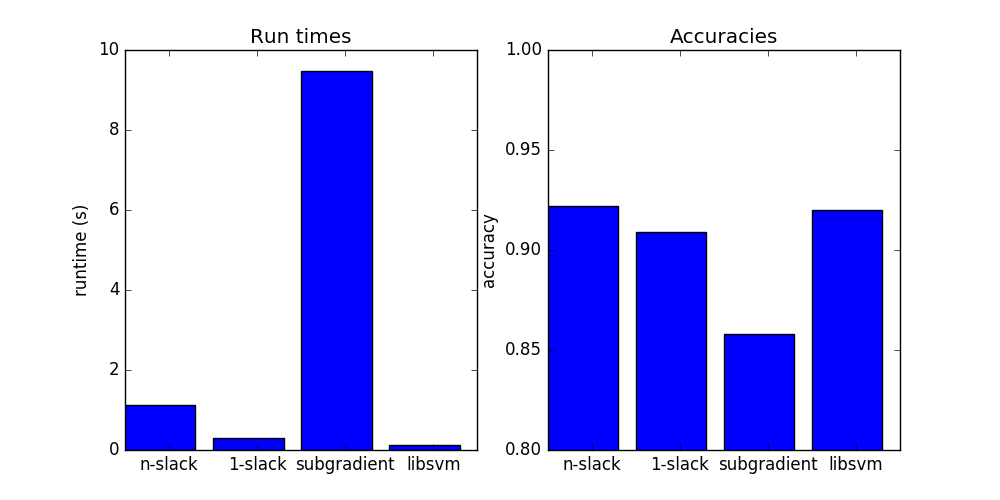# Binary SVM as SSVM¶

Example of training binary SVM using n-slack QP, 1-slack QP, SGD and SMO (libsvm). Our 1-slack QP does surprisingly well.

There are many parameters to tune and we can make 1-slack as good as the rest for the price of higher runtime, we can also try to make the others faster. We don’t really have a chance to beat LibSVM but that’s ok ;)Script output:

```Score with pystruct n-slack ssvm: 0.922222 (took 1.127200 seconds)
Score with pystruct 1-slack ssvm: 0.908889 (took 0.294215 seconds)
Score with pystruct subgradient ssvm: 0.857778 (took 9.471925 seconds)
Score with sklearn and libsvm: 0.920000 (took 0.116014 seconds)
```
```print(__doc__)

from time import time
import numpy as np
import matplotlib.pyplot as plt

from sklearn.cross_validation import train_test_split
from sklearn.svm import SVC

from pystruct.models import BinaryClf
from pystruct.learners import (NSlackSSVM, OneSlackSSVM,

# do a binary digit classification
X, y = digits.data, digits.target

# make binary task by doing odd vs even numers
y = y % 2
# code as +1 and -1
y = 2 * y - 1
X /= X.max()

X_train, X_test, y_train, y_test = train_test_split(X, y, random_state=0)

pbl = BinaryClf()
n_slack_svm = NSlackSSVM(pbl, C=10, batch_size=-1)
one_slack_svm = OneSlackSSVM(pbl, C=10, tol=0.1)
batch_size=10)

# we add a constant 1 feature for the bias
X_train_bias = np.hstack([X_train, np.ones((X_train.shape, 1))])
X_test_bias = np.hstack([X_test, np.ones((X_test.shape, 1))])

# n-slack cutting plane ssvm
start = time()
n_slack_svm.fit(X_train_bias, y_train)
time_n_slack_svm = time() - start
acc_n_slack = n_slack_svm.score(X_test_bias, y_test)
print("Score with pystruct n-slack ssvm: %f (took %f seconds)"
% (acc_n_slack, time_n_slack_svm))

## 1-slack cutting plane ssvm
start = time()
one_slack_svm.fit(X_train_bias, y_train)
time_one_slack_svm = time() - start
acc_one_slack = one_slack_svm.score(X_test_bias, y_test)
print("Score with pystruct 1-slack ssvm: %f (took %f seconds)"
% (acc_one_slack, time_one_slack_svm))

start = time()

print("Score with pystruct subgradient ssvm: %f (took %f seconds)"

libsvm = SVC(kernel='linear', C=10)
start = time()
libsvm.fit(X_train, y_train)
time_libsvm = time() - start
acc_libsvm = libsvm.score(X_test, y_test)
print("Score with sklearn and libsvm: %f (took %f seconds)"
% (acc_libsvm, time_libsvm))

# plot the results
fig, ax = plt.subplots(1, 2, figsize=(10, 5))
ax.bar(range(4), [time_n_slack_svm, time_one_slack_svm,
ax.set_xticks(np.arange(4) + .5)
ax.set_ylabel("runtime (s)")
ax.set_title("Run times")

ax.set_title("Accuracies")
ax.bar(range(4), [acc_n_slack, acc_one_slack,
Download Python source code: `plot_binary_svm.py`